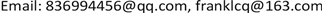MBR，中值滤波，多元线性回归算法，污泥龄, MBR Median Filtering Multiple Linear Regression Algorithm Sludge Age1. 引言

2. 使用线性回归算法和中值滤波分析算法2.1. 线性回归算法

2.1.1. 一元线性回归算法

2.1.2. 多元线性回归算法

y i = β 0 + β 1 x 1 + β 2 x 2 + ⋯ + β m − 1 x m − 1 + γ (1)

y ^ = b 0 + b 1 x i , 1 + b 2 x i , 2 + ⋯ + b m − 1 x i , m − 1 , i = 1 , 2 , ⋯ , n (2)

2.2. 中值滤波分析

2.2.1. 一维中值滤波

g j = medium   [ f j − r , f j − r + 1 , ⋯ , f j , f j + r ]

medium表示中值，即信号序列在模板中的排列顺序，取排在最中间的值，有 1 < j − r < j + r < N 。上式可通过滑动奇数长度的模块来实现。

2.2.2. 二维中值滤波

g ( x , y ) = medium x 0 , y 0 ∈ s   [ f ( x 0 , y 0 ) ]

2.3. 中值滤波分析去噪算法步骤

(1) 选取两层尺度，对含噪图像进行二维小波变换，得到高频子带和低频子带图像。

(2) 采用3 × 3窗口，单位距离为半径，对低频子带图像进行均值滤波。

w ¯ ( j , k ) = 1 L ∑ ( x , y ) ∈ A w ( x , y )

(3) 对各高频子带图像进行中值滤波。

(4) 对中值滤波后的高频子带图像进行阈值处理。

(5) 各高频子带图像在量化阈值时，也在进行系数增强。较大的增强系数在高尺度下的子带图像中选择，较小的增强系数在低尺度下的子带图像中选择，可由噪声与尺度变化的关系得到。

(6) 再对w(j, k)迸行小波逆变换，得到降噪后的输出图像。

3. 多元线性回归算法在MBR系统中对污泥龄的计算3.1. 多元线性回归算法对污泥龄的计算

θ x = HRT [ X + Y 0 ( c i − c s u p ) ] Y 0 ( c i − c e )

3.2. 计算结果分析

Comparison table of SRT simulation under stable state (30˚C

124.2922.890.057623.190.0453
214.7810.360.299015.310.0359
321.2719.030.105320.980.0136
414.5313.90.043414.210.022
511.4414.310.250910.990.0393
610.5411.930.131911.450.0863
715.513.640.1214.780.0465
86.937.990.15297.030.0144
928.4525.870.090727.010.0506
1021.2923.130.086421.810.0244

4. 中值滤波分析改进多元线性回归算法在MBR中对污泥龄的计算4.1. 用中值滤波分析来优化多元线性回归算法

4.2. 计算结果分析

5. 结论